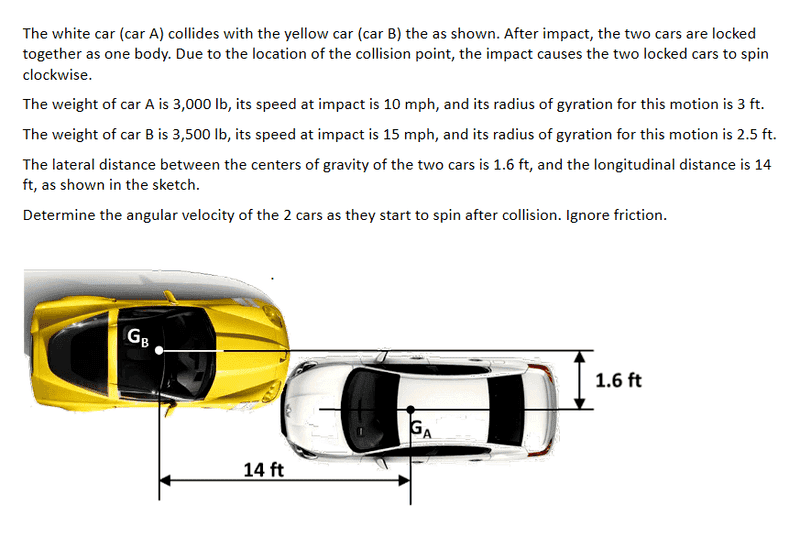# Conservation of linear & angular momentum head on collision

## Homework Statement

Two cars collide head on but offset from each others center of gravity. After the impact the two cars are locked together as one body. Due to the location of the collision point, the impact causes the two locked cars to spin clockwise.
Car A- traveling west going 10 mph, weighs 3000lb and the radius of gyration for this motion is 3 ft
Car B- traveling east going 15 mph, weighs 35000lb and the radius of gyration is 2.5 ft

The lateral distance between the center of gravity between the two cars is 1.6 ft and the longitudinal distance is 14 ft.
Determine the angular velocity of the 2 cars as they start to spin after the collision

## Homework Equations

I know that the linear and angular momentum is conserved and that kinetic energy is not. I am not sure how the linear momentum and kinetic energy is converted into the angular momentum because there is no angular momentum to begin with.
Any help or push in the right direction would help

## The Attempt at a SolutionBvU
Hello Ryan,